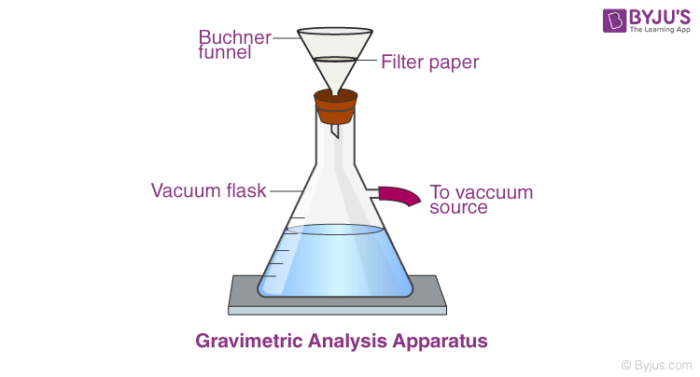# Gravimetric Analysis

## What is Gravimetric Analysis?

Gravimetric analysis is a method in analytical chemistry to determine the quantity of an analyte based on the mass of a solid. Example: Measuring the solids suspended in the water sample – Once a known volume of water is filtered, the collected solids are weighed.

### The principle of Gravimetric Analysis:

The principle behind the gravimetric analysis is that the mass of an ion in a pure compound and can be determined. Later, used to find the mass percent of the same ion in a known quantity of an impure compound.Gravimetric Analysis Apparatus

## Steps followed in the Gravimetric Analysis

1. Preparation of a solution containing a known weight of the sample.
2. Separation of the desired constituent.
3. Weighing the isolated constituent.
4. Computation of the amount of the particular constituent in the sample from the observed weight of the isolated substance.

## Types of Gravimetric Analysis

There are 4 fundamental types of gravimetric analysis. Of which, there are 2 common types involving changes in the phase of the analyte to separate it from the rest of a mixture, resulting in a change in mass.

#### Volatilisation gravimetry

• Volatilisation Gravimetry involves separating components of our mixture by heating or chemically decomposing the sample.

#### Precipitation gravimetry

• Precipitation Gravimetry uses a precipitation reaction to separate one or more parts of a solution by incorporating it into a solid.

#### Electrogravimetry

• Electrogravimetry is a method used to separate and quantify ions of a substance, usually a metal.

#### Thermogravimetry

• Thermogravimetry is a method of thermal analysis in which changes in physical and chemical properties of materials are measured as a function of increasing temperature or as a function of time.

If the methods are followed carefully, it provides exceedingly precise analysis. It is used to determine the atomic masses of many elements to six-figure accuracy. It provides little room for instrumental error and does not require a series of standards for calculation of an unknown.

It usually provides only for the analysis of a single element, or a limited group of elements, at a time. Comparing modern dynamic flash combustion coupled with gas chromatography with traditional combustion analysis.

## Examples of Gravimetric Analysis

#### Question:

An ore is analysed for the manganese content by converting the manganese to Mn3O4 and weighing it. If a 1.52g sample yields Mn3O4 weighing 0.126g, what would be the percent Mn3O3 in the sample? The percent of Mn?

#### Solution

Mn3O4 consists of Mn2O3 and MnO
All the manganese in the ore is converted into Mn3O4
Amount of Mn in Mn3O4 = (0.126/2.29) × (3 × 55)
= 0.091 g
% of Mn in the ore = (0.091/1.52 ) × 100
= 5.973 %
% ofMn2O3 = [0.126g Mn3O4 × (3 Mn2O3 / 3 Mn3O4) (Mn2O3 / Mn3O4 ) × 100 ]/ 1.52g sample
= 8.58 %
%Mn = [0.126g Mn3O4 × (3 Mn /Mn3O4) (gMn/Mn3O4) × 100] / 1.52g sample
={0.126g×[3(54.94)/228.8]1.52g}×100%
=5.97%

## Frequently Asked Questions – FAQs

### What is the principle of gravimetric analysis?

Gravimetric analysis relies on the contrast of the masses of two analyte-containing compounds. The idea behind gravimetric analysis is that it is possible to calculate the mass of an ion in a pure compound and then use it to calculate the mass percentage of the same ion in a specified volume of an impure compound.

### What are the applications of gravimetric analysis?

In analytical chemistry, gravimetric analysis is a way of determining the analyte quantity based on the density of a solid. Example: Measuring the solids suspended in the water sample. The collected solids are weighed until a known water volume is purified.

### What is the difference between gravimetric and volumetric analysis?

A gravimetric feeder is a self-calibrating, speed-based dosing device based on weight. On the other hand, a volumetric feeder does this depending on the velocity of volume. Using loss-in-weight technique, which calculates the decreased weight during dosing, weight is estimated.

### How does volumetric titration work?

A widely-used quantitative computational methodology is volumetric analysis. This approach requires the calculation of the volume of a solution of known concentration that is used to assess the analyte concentration, as the name suggests. The end point of the titration is the point at which the colour alteration takes place.

### What are the advantages of gravimetric analysis?

Gravimetric analysis allows for highly detailed analysis if procedures are practised closely. In fact, to calculate the atomic masses of several elements in the periodic table to six figure precision, gravimetric analysis was used.

Test your knowledge on Gravimetric Analysis